# MCQs on Kirchhoffs Law

Kirchhoff’s junction Law or Kirchhoff’s Current Law

The total current entering a junction or a node is equal to the charge leaving the node as no charge is lost.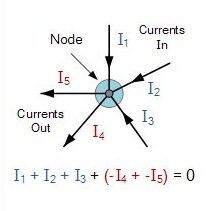Kirchhoff’s Loop Law or Kirchhoff’s Voltage Law

The voltage around a loop equals the sum of every voltage drop in the same loop for any closed network and also equals zero.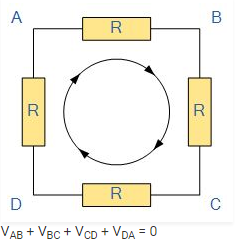Q1: Calculate the voltage across the 5ohm resistor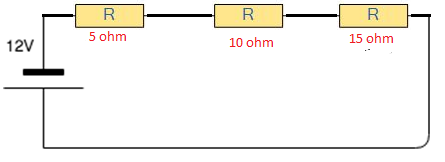1. 12 V
2. 2 V
3. 10 V
4. 0 V

Q2: Which basic law should be followed to analyse the circuit?

1. Newton’s law
3. Amperes law
4. Kirchoff’s law

Q3: Calculate the value of V1 and V2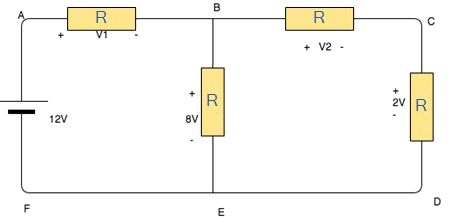1. 4V, 6V
2. 5V,6V
3. 6V, 7V
4. 7V, 8V

Q4: In Kirchhoff’s first law Σi = 0 at the junction is based on the conservation of

1. Energy
2. Charge
3. Momentum
4. Speed

Q5: In the circuit shown below what will be the reading in the voltmeter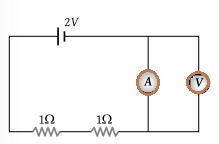1. 2 V
2. 1 V
3. 0.5 V
4. Zero

Q6: What is the relation between currents in the figure below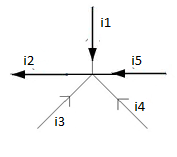1. i2 = i1 + i3 + i4 +i5
2. i2 – i1 = i3 – i4 +i5
3. i3 +i4 =i1+i2+i5
4. I1+i5 =i2+i3+i4

Answer: (a) i2 = i1 + i3 + i4 +i5

Q7: The algebraic sum of voltages around any closed path in a network is equal to

1. Infinity
2. 1
3. 0
4. Negative polarity

Q8: Kirchhoff’s Current Law is based on

1. The charge can be accumulated at the node
2. Charge cannot be accumulated at the node
3. Energy is stored at the node
4. Depending on the circuit charge can be accumulated at the circuit

Answer: (b) Charge cannot be accumulated at the node

Q9: The terminal potential difference will be greater than its emf when it is

1. In open circuit
2. Being charged
3. Being charged or discharged
4. Being discharged